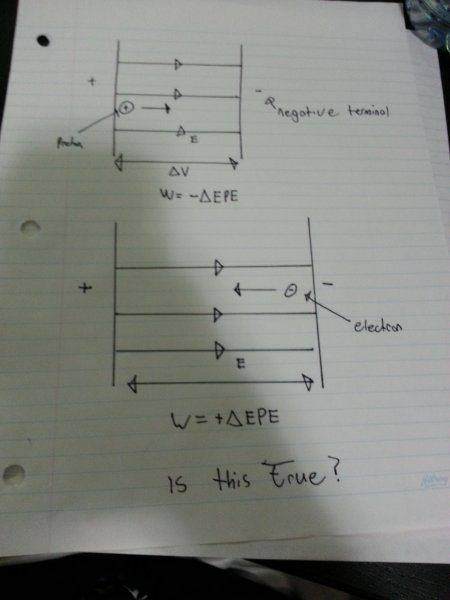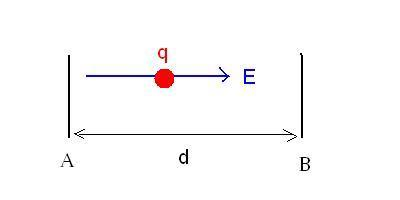# When does work equal negative electric potential energy

• sanhuy

## Homework Statement

Let EPE be change in electric potential energy

so if a positive charge is moving towards the negative terminal with the electric field it would mean that it is losing PE therefore work = - EPE, and if a negative charge is moving against the electric field towards the positive terminal it would gain potential energy? therefore W = + Delta EPE

can someone confirm if this concept is right and i should use it for life.

## Homework Equations

W = EPE = q*DELTA V

## The Attempt at a SolutionYes, you are right.

Let we have capacitor plates A and B d distance apart. Let be the potential UA at A (on the left) and UB at B and UA>UB. The electric field is E=(UA-UB)/d, and points to the right, away from the positive plate. The force acting on charge q is F= qE, and the work done by the field when q moves from A to B is W=q(UA-UB). We define change of potential by Ufinal -Uinitial, and the change of potential energy is ΔEPE=q(Ufinal -Uinitial). Uinitial=UA, Ufinal=UB , so ΔEPE=q(UB-UA) which is opposite to the work done by the electric field. W = -ΔEPE.Last edited: